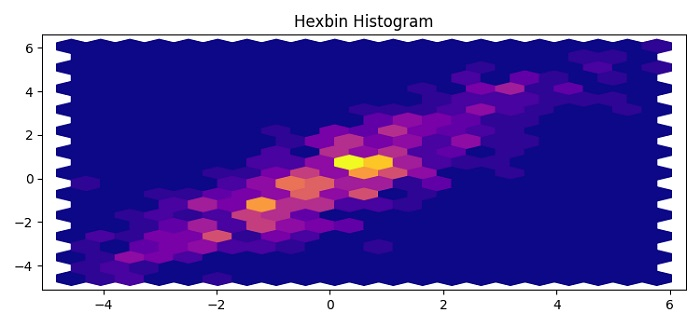# How to plot hexbin histogram in Matplotlib?

To plot a hexbin histogram in matplotlib, we can take the following steps −

• Set the figure size and adjust the padding between and around the subplots.

• Create x and y data points using numpy.

• Create a figure and a set of subplots.

• Plot x and y using hexbin() method.

• Set the title of the plot.

• To display the figure, use show() method.

## Example

import numpy as np
from matplotlib import pyplot as plt

plt.rcParams["figure.figsize"] = [7.50, 3.50]
plt.rcParams["figure.autolayout"] = True

x = 2 * np.random.randn(5000)
y = x + np.random.randn(5000)

fig, ax = plt.subplots()
_ = ax.hexbin(x[::10], y[::10], gridsize=20, cmap='plasma')

ax.set_title('Hexbin Histogram')

plt.show()

## OutputUpdated on: 03-Jun-2021

286 Views